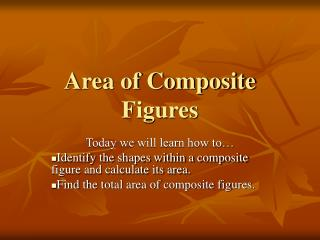DownloadDownload PresentationArea of Composite Figures

# Area of Composite Figures

Download Presentation## Area of Composite Figures

- - - - - - - - - - - - - - - - - - - - - - - - - - - E N D - - - - - - - - - - - - - - - - - - - - - - - - - - -
##### Presentation Transcript

1. Area of Composite Figures Today we will learn how to… Identify the shapes within a composite figure and calculate its area. Find the total area of composite figures.

2. Things we must know… • How to find the area of… • Squares • A = s2 • Triangles • A = ½ b h • Rectangles • A = l w • Trapezoids • A = ½ h(b1 + b2) • Circles • A = п r2

3. Steps to finding area of composite figures • Identify the shapes that make up the composite figure • Calculate the area of each figure • Add or Subtract to find the total area of the composite figure

4. Examples • Easy • Medium • Not so bad • Challenging